# 3D 컨볼루션을 사용한 비디오 동화 작업

Yunpeng Li, Dominik Roblek, and Marco Tagliasacchi. From Here to There: Video Inbetweening Using Direct 3D Convolutions, 2019.

https://arxiv.org/abs/1905.10240

현재 Hub의 특징:

• BAIR 로봇 푸싱 비디오 및 KTH 액션 비디오 데이터세트에 대한 모델이 있습니다(이 colab에서는 BAIR만 사용).
• Hub에서 이미 BAIR 데이터세트를 사용할 수 있습니다. 그러나 KTH 비디오는 사용자가 직접 제공해야 합니다.
• 지금은 평가(비디오 생성)만 가능합니다.
• 배치 크기와 프레임 크기는 하드 코딩됩니다.

## 설정

tfds.load('bair_robot_pushing_small', split='test')는 훈련 데이터도 포함하는 30GB 아카이브를 다운로드하므로 190MB 테스트 데이터만 포함하는 별도의 아카이브를 다운로드합니다. 사용된 데이터세트는 이 논문에 게시되었고 Creative Commons BY 4.0으로 라이선스가 부여되었습니다.

import tensorflow as tf

import matplotlib.pyplot as plt
import numpy as np
import seaborn as sns
import tensorflow_hub as hub
import tensorflow_datasets as tfds

from tensorflow_datasets.core import SplitGenerator
from tensorflow_datasets.video.bair_robot_pushing import BairRobotPushingSmall

import tempfile
import pathlib

TEST_DIR = pathlib.Path(tempfile.mkdtemp()) / "bair_robot_pushing_small/softmotion30_44k/test/"

# Download the test split to $TEST_DIR mkdir -p$TEST_DIR
wget -nv https://storage.googleapis.com/download.tensorflow.org/data/bair_test_traj_0_to_255.tfrecords -O \$TEST_DIR/traj_0_to_255.tfrecords

2020-11-12 02:15:03 URL:https://storage.googleapis.com/download.tensorflow.org/data/bair_test_traj_0_to_255.tfrecords [189852160/189852160] -> "/tmp/tmp1kks8s3s/bair_robot_pushing_small/softmotion30_44k/test/traj_0_to_255.tfrecords" 

# Since the dataset builder expects the train and test split to be downloaded,
# patch it so it only expects the test data to be available
builder = BairRobotPushingSmall()
test_generator = SplitGenerator(name='test', gen_kwargs={"filedir": str(TEST_DIR)})
builder._split_generators = lambda _: [test_generator]

Downloading and preparing dataset bair_robot_pushing_small/2.0.0 (download: 30.06 GiB, generated: Unknown size, total: 30.06 GiB) to /home/kbuilder/tensorflow_datasets/bair_robot_pushing_small/2.0.0...
WARNING:tensorflow:From /home/kbuilder/.local/lib/python3.6/site-packages/tensorflow_datasets/video/bair_robot_pushing.py:103: tf_record_iterator (from tensorflow.python.lib.io.tf_record) is deprecated and will be removed in a future version.
Instructions for updating:
Use eager execution and:
tf.data.TFRecordDataset(path)
WARNING:tensorflow:From /home/kbuilder/.local/lib/python3.6/site-packages/tensorflow_datasets/video/bair_robot_pushing.py:103: tf_record_iterator (from tensorflow.python.lib.io.tf_record) is deprecated and will be removed in a future version.
Instructions for updating:
Use eager execution and:
tf.data.TFRecordDataset(path)
Shuffling and writing examples to /home/kbuilder/tensorflow_datasets/bair_robot_pushing_small/2.0.0.incompleteOGMHWO/bair_robot_pushing_small-test.tfrecord
Dataset bair_robot_pushing_small downloaded and prepared to /home/kbuilder/tensorflow_datasets/bair_robot_pushing_small/2.0.0. Subsequent calls will reuse this data.


## BAIR: numpy 배열 입력에 기초한 데모

WARNING:tensorflow:From /home/kbuilder/.local/lib/python3.6/site-packages/tensorflow_datasets/core/features/feature.py:425: calling map_fn_v2 (from tensorflow.python.ops.map_fn) with back_prop=False is deprecated and will be removed in a future version.
Instructions for updating:
results = tf.map_fn(fn, elems, back_prop=False)
Use:
WARNING:tensorflow:From /home/kbuilder/.local/lib/python3.6/site-packages/tensorflow_datasets/core/features/feature.py:425: calling map_fn_v2 (from tensorflow.python.ops.map_fn) with back_prop=False is deprecated and will be removed in a future version.
Instructions for updating:
results = tf.map_fn(fn, elems, back_prop=False)
Use:


Test videos shape [batch_size, start/end frame, height, width, num_channels]:  (16, 2, 64, 64, 3)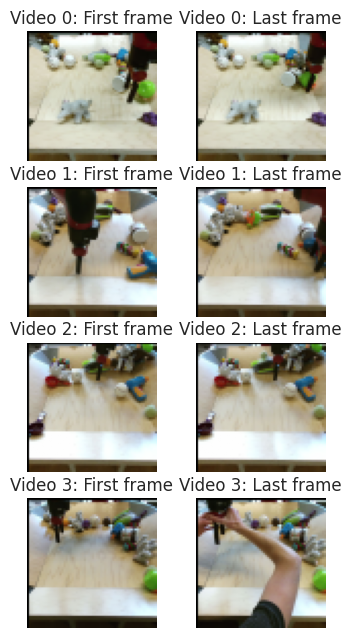### Hub 모듈 로드하기

hub_handle = 'https://tfhub.dev/google/tweening_conv3d_bair/1'

WARNING:tensorflow:Unable to create a python object for variable <tf.Variable 'video_discriminator/conv_0/weights:0' shape=(4, 4, 4, 3, 64) dtype=float32_ref> because it is a reference variable. It may not be visible to training APIs. If this is a problem, consider rebuilding the SavedModel after running tf.compat.v1.enable_resource_variables().
WARNING:tensorflow:Unable to create a python object for variable <tf.Variable 'video_discriminator/conv_0/weights:0' shape=(4, 4, 4, 3, 64) dtype=float32_ref> because it is a reference variable. It may not be visible to training APIs. If this is a problem, consider rebuilding the SavedModel after running tf.compat.v1.enable_resource_variables().
WARNING:tensorflow:Unable to create a python object for variable <tf.Variable 'video_discriminator/conv_0/LayerNorm/beta:0' shape=(64,) dtype=float32_ref> because it is a reference variable. It may not be visible to training APIs. If this is a problem, consider rebuilding the SavedModel after running tf.compat.v1.enable_resource_variables().
WARNING:tensorflow:Unable to create a python object for variable <tf.Variable 'video_discriminator/conv_0/LayerNorm/beta:0' shape=(64,) dtype=float32_ref> because it is a reference variable. It may not be visible to training APIs. If this is a problem, consider rebuilding the SavedModel after running tf.compat.v1.enable_resource_variables().
WARNING:tensorflow:Unable to create a python object for variable <tf.Variable 'video_discriminator/conv_0/LayerNorm/gamma:0' shape=(64,) dtype=float32_ref> because it is a reference variable. It may not be visible to training APIs. If this is a problem, consider rebuilding the SavedModel after running tf.compat.v1.enable_resource_variables().
WARNING:tensorflow:Unable to create a python object for variable <tf.Variable 'video_discriminator/conv_0/LayerNorm/gamma:0' shape=(64,) dtype=float32_ref> because it is a reference variable. It may not be visible to training APIs. If this is a problem, consider rebuilding the SavedModel after running tf.compat.v1.enable_resource_variables().
WARNING:tensorflow:Unable to create a python object for variable <tf.Variable 'video_discriminator/conv_1/weights:0' shape=(4, 4, 4, 64, 128) dtype=float32_ref> because it is a reference variable. It may not be visible to training APIs. If this is a problem, consider rebuilding the SavedModel after running tf.compat.v1.enable_resource_variables().
WARNING:tensorflow:Unable to create a python object for variable <tf.Variable 'video_discriminator/conv_1/weights:0' shape=(4, 4, 4, 64, 128) dtype=float32_ref> because it is a reference variable. It may not be visible to training APIs. If this is a problem, consider rebuilding the SavedModel after running tf.compat.v1.enable_resource_variables().
WARNING:tensorflow:Unable to create a python object for variable <tf.Variable 'video_discriminator/conv_1/LayerNorm/beta:0' shape=(128,) dtype=float32_ref> because it is a reference variable. It may not be visible to training APIs. If this is a problem, consider rebuilding the SavedModel after running tf.compat.v1.enable_resource_variables().
WARNING:tensorflow:Unable to create a python object for variable <tf.Variable 'video_discriminator/conv_1/LayerNorm/beta:0' shape=(128,) dtype=float32_ref> because it is a reference variable. It may not be visible to training APIs. If this is a problem, consider rebuilding the SavedModel after running tf.compat.v1.enable_resource_variables().
WARNING:tensorflow:Unable to create a python object for variable <tf.Variable 'video_discriminator/conv_0/weights:0' shape=(4, 4, 4, 3, 64) dtype=float32_ref> because it is a reference variable. It may not be visible to training APIs. If this is a problem, consider rebuilding the SavedModel after running tf.compat.v1.enable_resource_variables().
WARNING:tensorflow:Unable to create a python object for variable <tf.Variable 'video_discriminator/conv_0/weights:0' shape=(4, 4, 4, 3, 64) dtype=float32_ref> because it is a reference variable. It may not be visible to training APIs. If this is a problem, consider rebuilding the SavedModel after running tf.compat.v1.enable_resource_variables().
WARNING:tensorflow:Unable to create a python object for variable <tf.Variable 'video_discriminator/conv_0/LayerNorm/beta:0' shape=(64,) dtype=float32_ref> because it is a reference variable. It may not be visible to training APIs. If this is a problem, consider rebuilding the SavedModel after running tf.compat.v1.enable_resource_variables().
WARNING:tensorflow:Unable to create a python object for variable <tf.Variable 'video_discriminator/conv_0/LayerNorm/beta:0' shape=(64,) dtype=float32_ref> because it is a reference variable. It may not be visible to training APIs. If this is a problem, consider rebuilding the SavedModel after running tf.compat.v1.enable_resource_variables().
WARNING:tensorflow:Unable to create a python object for variable <tf.Variable 'video_discriminator/conv_0/LayerNorm/gamma:0' shape=(64,) dtype=float32_ref> because it is a reference variable. It may not be visible to training APIs. If this is a problem, consider rebuilding the SavedModel after running tf.compat.v1.enable_resource_variables().
WARNING:tensorflow:Unable to create a python object for variable <tf.Variable 'video_discriminator/conv_0/LayerNorm/gamma:0' shape=(64,) dtype=float32_ref> because it is a reference variable. It may not be visible to training APIs. If this is a problem, consider rebuilding the SavedModel after running tf.compat.v1.enable_resource_variables().
WARNING:tensorflow:Unable to create a python object for variable <tf.Variable 'video_discriminator/conv_1/weights:0' shape=(4, 4, 4, 64, 128) dtype=float32_ref> because it is a reference variable. It may not be visible to training APIs. If this is a problem, consider rebuilding the SavedModel after running tf.compat.v1.enable_resource_variables().
WARNING:tensorflow:Unable to create a python object for variable <tf.Variable 'video_discriminator/conv_1/weights:0' shape=(4, 4, 4, 64, 128) dtype=float32_ref> because it is a reference variable. It may not be visible to training APIs. If this is a problem, consider rebuilding the SavedModel after running tf.compat.v1.enable_resource_variables().
WARNING:tensorflow:Unable to create a python object for variable <tf.Variable 'video_discriminator/conv_1/LayerNorm/beta:0' shape=(128,) dtype=float32_ref> because it is a reference variable. It may not be visible to training APIs. If this is a problem, consider rebuilding the SavedModel after running tf.compat.v1.enable_resource_variables().
WARNING:tensorflow:Unable to create a python object for variable <tf.Variable 'video_discriminator/conv_1/LayerNorm/beta:0' shape=(128,) dtype=float32_ref> because it is a reference variable. It may not be visible to training APIs. If this is a problem, consider rebuilding the SavedModel after running tf.compat.v1.enable_resource_variables().
WARNING:tensorflow:Unable to create a python object for variable <tf.Variable 'video_discriminator/conv_0/weights:0' shape=(4, 4, 4, 3, 64) dtype=float32_ref> because it is a reference variable. It may not be visible to training APIs. If this is a problem, consider rebuilding the SavedModel after running tf.compat.v1.enable_resource_variables().
WARNING:tensorflow:Unable to create a python object for variable <tf.Variable 'video_discriminator/conv_0/weights:0' shape=(4, 4, 4, 3, 64) dtype=float32_ref> because it is a reference variable. It may not be visible to training APIs. If this is a problem, consider rebuilding the SavedModel after running tf.compat.v1.enable_resource_variables().
WARNING:tensorflow:Unable to create a python object for variable <tf.Variable 'video_discriminator/conv_0/LayerNorm/beta:0' shape=(64,) dtype=float32_ref> because it is a reference variable. It may not be visible to training APIs. If this is a problem, consider rebuilding the SavedModel after running tf.compat.v1.enable_resource_variables().
WARNING:tensorflow:Unable to create a python object for variable <tf.Variable 'video_discriminator/conv_0/LayerNorm/beta:0' shape=(64,) dtype=float32_ref> because it is a reference variable. It may not be visible to training APIs. If this is a problem, consider rebuilding the SavedModel after running tf.compat.v1.enable_resource_variables().
WARNING:tensorflow:Unable to create a python object for variable <tf.Variable 'video_discriminator/conv_0/LayerNorm/gamma:0' shape=(64,) dtype=float32_ref> because it is a reference variable. It may not be visible to training APIs. If this is a problem, consider rebuilding the SavedModel after running tf.compat.v1.enable_resource_variables().
WARNING:tensorflow:Unable to create a python object for variable <tf.Variable 'video_discriminator/conv_0/LayerNorm/gamma:0' shape=(64,) dtype=float32_ref> because it is a reference variable. It may not be visible to training APIs. If this is a problem, consider rebuilding the SavedModel after running tf.compat.v1.enable_resource_variables().
WARNING:tensorflow:Unable to create a python object for variable <tf.Variable 'video_discriminator/conv_1/weights:0' shape=(4, 4, 4, 64, 128) dtype=float32_ref> because it is a reference variable. It may not be visible to training APIs. If this is a problem, consider rebuilding the SavedModel after running tf.compat.v1.enable_resource_variables().
WARNING:tensorflow:Unable to create a python object for variable <tf.Variable 'video_discriminator/conv_1/weights:0' shape=(4, 4, 4, 64, 128) dtype=float32_ref> because it is a reference variable. It may not be visible to training APIs. If this is a problem, consider rebuilding the SavedModel after running tf.compat.v1.enable_resource_variables().
WARNING:tensorflow:Unable to create a python object for variable <tf.Variable 'video_discriminator/conv_1/LayerNorm/beta:0' shape=(128,) dtype=float32_ref> because it is a reference variable. It may not be visible to training APIs. If this is a problem, consider rebuilding the SavedModel after running tf.compat.v1.enable_resource_variables().
WARNING:tensorflow:Unable to create a python object for variable <tf.Variable 'video_discriminator/conv_1/LayerNorm/beta:0' shape=(128,) dtype=float32_ref> because it is a reference variable. It may not be visible to training APIs. If this is a problem, consider rebuilding the SavedModel after running tf.compat.v1.enable_resource_variables().
WARNING:tensorflow:Unable to create a python object for variable <tf.Variable 'video_discriminator/conv_0/weights:0' shape=(4, 4, 4, 3, 64) dtype=float32_ref> because it is a reference variable. It may not be visible to training APIs. If this is a problem, consider rebuilding the SavedModel after running tf.compat.v1.enable_resource_variables().
WARNING:tensorflow:Unable to create a python object for variable <tf.Variable 'video_discriminator/conv_0/weights:0' shape=(4, 4, 4, 3, 64) dtype=float32_ref> because it is a reference variable. It may not be visible to training APIs. If this is a problem, consider rebuilding the SavedModel after running tf.compat.v1.enable_resource_variables().
WARNING:tensorflow:Unable to create a python object for variable <tf.Variable 'video_discriminator/conv_0/LayerNorm/beta:0' shape=(64,) dtype=float32_ref> because it is a reference variable. It may not be visible to training APIs. If this is a problem, consider rebuilding the SavedModel after running tf.compat.v1.enable_resource_variables().
WARNING:tensorflow:Unable to create a python object for variable <tf.Variable 'video_discriminator/conv_0/LayerNorm/beta:0' shape=(64,) dtype=float32_ref> because it is a reference variable. It may not be visible to training APIs. If this is a problem, consider rebuilding the SavedModel after running tf.compat.v1.enable_resource_variables().
WARNING:tensorflow:Unable to create a python object for variable <tf.Variable 'video_discriminator/conv_0/LayerNorm/gamma:0' shape=(64,) dtype=float32_ref> because it is a reference variable. It may not be visible to training APIs. If this is a problem, consider rebuilding the SavedModel after running tf.compat.v1.enable_resource_variables().
WARNING:tensorflow:Unable to create a python object for variable <tf.Variable 'video_discriminator/conv_0/LayerNorm/gamma:0' shape=(64,) dtype=float32_ref> because it is a reference variable. It may not be visible to training APIs. If this is a problem, consider rebuilding the SavedModel after running tf.compat.v1.enable_resource_variables().
WARNING:tensorflow:Unable to create a python object for variable <tf.Variable 'video_discriminator/conv_1/weights:0' shape=(4, 4, 4, 64, 128) dtype=float32_ref> because it is a reference variable. It may not be visible to training APIs. If this is a problem, consider rebuilding the SavedModel after running tf.compat.v1.enable_resource_variables().
WARNING:tensorflow:Unable to create a python object for variable <tf.Variable 'video_discriminator/conv_1/weights:0' shape=(4, 4, 4, 64, 128) dtype=float32_ref> because it is a reference variable. It may not be visible to training APIs. If this is a problem, consider rebuilding the SavedModel after running tf.compat.v1.enable_resource_variables().
WARNING:tensorflow:Unable to create a python object for variable <tf.Variable 'video_discriminator/conv_1/LayerNorm/beta:0' shape=(128,) dtype=float32_ref> because it is a reference variable. It may not be visible to training APIs. If this is a problem, consider rebuilding the SavedModel after running tf.compat.v1.enable_resource_variables().
WARNING:tensorflow:Unable to create a python object for variable <tf.Variable 'video_discriminator/conv_1/LayerNorm/beta:0' shape=(128,) dtype=float32_ref> because it is a reference variable. It may not be visible to training APIs. If this is a problem, consider rebuilding the SavedModel after running tf.compat.v1.enable_resource_variables().


### 비디오 생성 및 표시하기

filled_frames = module(input_frames)['default'] / 255.0

# Show sequences of generated video frames.

# Concatenate start/end frames and the generated filled frames for the new videos.
generated_videos = np.concatenate([input_frames[:, :1] / 255.0, filled_frames, input_frames[:, 1:] / 255.0], axis=1)

for video_id in range(4):
fig = plt.figure(figsize=(10 * 2, 2))
for frame_id in range(1, 16):
ax = fig.add_axes([frame_id * 1 / 16., 0, (frame_id + 1) * 1 / 16., 1],
xmargin=0, ymargin=0)
ax.imshow(generated_videos[video_id, frame_id])
ax.axis('off')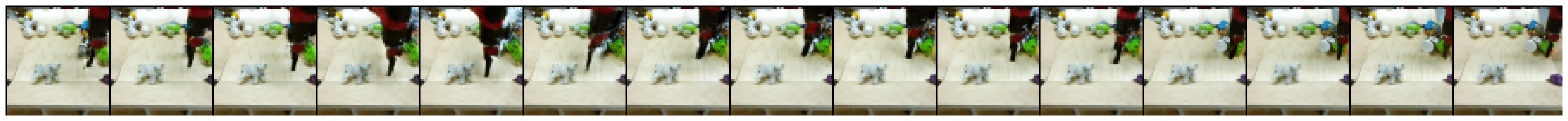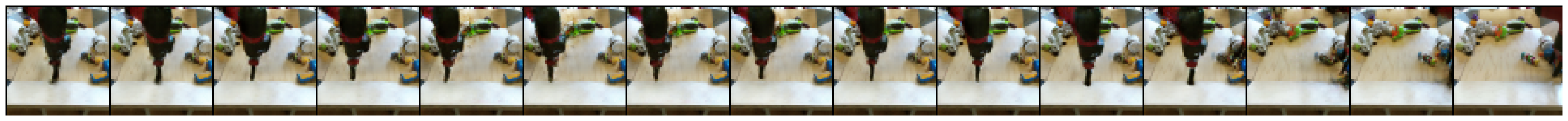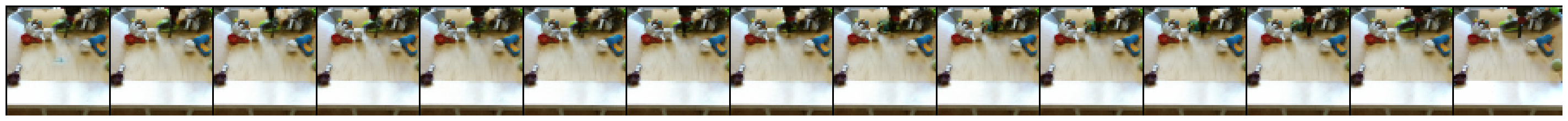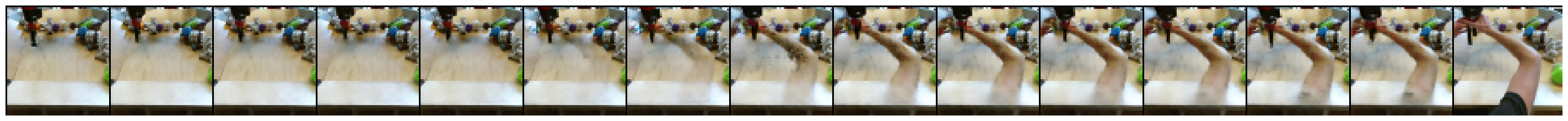[{ "type": "thumb-down", "id": "missingTheInformationINeed", "label":"필요한 정보가 없음" },{ "type": "thumb-down", "id": "tooComplicatedTooManySteps", "label":"너무 복잡함/단계 수가 너무 많음" },{ "type": "thumb-down", "id": "outOfDate", "label":"오래됨" },{ "type": "thumb-down", "id": "translationIssue", "label":"번역 문제" },{ "type": "thumb-down", "id": "samplesCodeIssue", "label":"Samples / code issue" },{ "type": "thumb-down", "id": "otherDown", "label":"기타" }]
[{ "type": "thumb-up", "id": "easyToUnderstand", "label":"이해하기 쉬움" },{ "type": "thumb-up", "id": "solvedMyProblem", "label":"문제가 해결됨" },{ "type": "thumb-up", "id": "otherUp", "label":"기타" }]